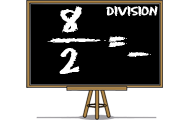# NumbersFiltering on knowledge field: Numbers

Math games that will teach you about mathematics, numbers and counting. Practice the basic number perception with an abacus, or practice the four rules of arithmetic. You will learn math in a joyful way by playing these games. Here we have collected all the games on the subject Math. The games suit preschool, elementary school and all the way up to high school. The games may be sorted by popularity, target group, release date or name.
0%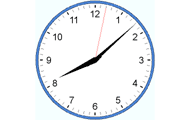## Tell time in English

• Clock
• Analog clock
• Numbers
Practice analog clock. Practice the basics such as full hours, half-hours, quarters and minutes. Watch the three clocks and choose the clock that match the requested time.
0%## Number bonds

• Numbers
• Mental calculation
• Number bonds
Try to find the number that is a number bond to another number and adds up to the given sum. Practice part-part-whole relationships to the numbers 0-20 and facilitates the important mental calculation
0%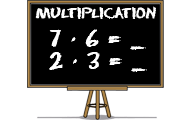## Multiplication

• Numbers
• Multiplication
• Mental calculation
Learn multiplication with this math game for students. Find the product of the factors in all times tables.
0%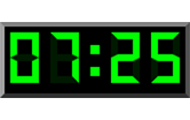## Digital clock in English

• Clock
• Digital clock
• Numbers
Practice the digital clock with 24 hours. Read a written time and find the corresponding time among the three digital clocks.
0%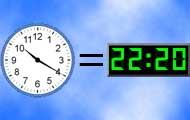## Match clocks

• Clock
• Analog clock
• Digital clock
• Numbers
Match clocks that can be both analog and digital. Practice telling time with both types of clocks.
0%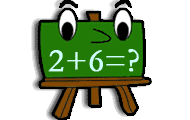• Numbers
• Mental calculation
Practice addition in math game with several levels of increasing difficulty. Add two numbers and find the correct sum.
0%## Writing numbers in words

• Numbers
• Digits
• Words
• Write
Practice how numbers are written in words. In English and both numbers to words and words to numbers.
0%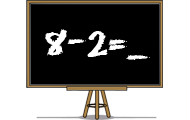## Subtraction

• Numbers
• Subtraction
• Mental calculation
Practice subtraction or minus in a skill training game. Find the difference between two numbers in different levels in this math game.
0%